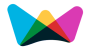#Lumpsum Investment Return | Final Maturity Value(FMV) Calculator#Lump-Sum Calculator

An online finance tool to calculate the final maturity value(FMV) of a single deposit or lump-sum investment.

%
Step by Step calculation# Lumpsum Formula

This final maturity value (FMV) calculator can be used to calculate the future value (FV) or final maturity of an investment with given inputs of Lumpsump deposit (P), interest (I) and yield rate (R).

Final Maturity Value Formula (FMV) = P * (1 + r / n)n*t

Where,

Lumpsum Amount or Investment Amount (P) is the present value or principal amount to be invested.

Duration (or) Total Number of Periods (t) is the total number of compounding periods for the life of the investment.

Rate of Return or Interest Rate (r) is the interest rate per compounding period.

Compounding (n) is the number of times compounding occurs per period. If a period is a year then annually=1, quarterly=4, monthly=12, daily = 365.

In this calculator, we used the monthly=12 (n=12)

Final Maturity Value (FMV) is the calculated future value of the investment.

### Final Maturity Value (FMV)

The final maturity value(FMV) or future value calculator can be used to determine future value, or FMV, or FV, in financing. FMV is simply what money is expected to be worth in the future.

Typically, cash in a savings account or a hold in a bond purchase earns compound interest and so has a different value in the future.

## Lumpsum Examples

A person is investing \$1,000 at a 5 percent interest rate, compounded quarterly for five years.

In this example, the compound interest calculation would be: FMV = (1000 (1 + .05/4))4(5)

FMV = (1000 (1 + .05/4))4(5)

= \$1,282.04